Download Presentation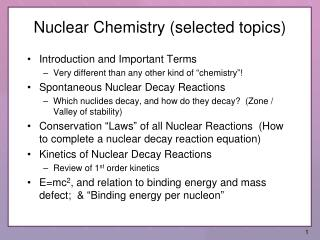Nuclear Chemistry (selected topics)

# Nuclear Chemistry (selected topics) - PowerPoint PPT Presentation

Nuclear Chemistry (selected topics). Introduction and Important Terms Very different than any other kind of “chemistry”! Spontaneous Nuclear Decay Reactions Which nuclides decay, and how do they decay? (Zone / Valley of stability)I am the owner, or an agent authorized to act on behalf of the owner, of the copyrighted work described.
Download Presentation## Nuclear Chemistry (selected topics)

An Image/Link below is provided (as is) to download presentation

Download Policy: Content on the Website is provided to you AS IS for your information and personal use and may not be sold / licensed / shared on other websites without getting consent from its author.While downloading, if for some reason you are not able to download a presentation, the publisher may have deleted the file from their server.

- - - - - - - - - - - - - - - - - - - - - - - - - - E N D - - - - - - - - - - - - - - - - - - - - - - - - - -
Presentation Transcript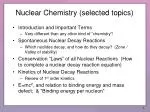Nuclear Chemistry (selected topics)
• Introduction and Important Terms
• Very different than any other kind of “chemistry”!
• Spontaneous Nuclear Decay Reactions
• Which nuclides decay, and how do they decay? (Zone / Valley of stability)
• Conservation “Laws” of all Nuclear Reactions (How to complete a nuclear decay reaction equation)
• Kinetics of Nuclear Decay Reactions
• Review of 1st order kinetics
• E=mc2, and relation to binding energy and mass defect; & “Binding energy per nucleon”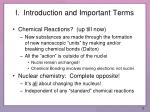I. Introduction and Important Terms
• Chemical Reactions? (up till now)
• New substances are made through the formation of new nanoscopic “units” by making and/or breaking chemical bonds (Dalton)
• All the “action” is outside of the nuclei
• Nuclei remain unchanged!
• Chemical Bonding involves moving electrons, not nuclei
• Nuclear chemistry: Complete opposite!
• It’s all about changing the nucleus!
• Independent of any “standard” chemical reactions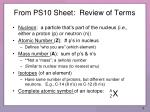From PS10 Sheet: Review of Terms

X

A

Z

• Nucleon: a particle that’s part of the nucleus (i.e., either a proton (p) or neutron (n))
• Atomic Number (Z): # p’s in nucleus
• Defines “who you are” (which element)
• Mass number (A): sum of p’s & n’s
• **Not a “mass”; a (whole) number
• Similar to nuclear mass (to nearest amu)
• Isotopes (of an element)
• Have same number of protons, but different number of neutrons. E.g., C-14 (8 n’s) vs C-12 (6 n’s)
• Complete atomic symbol of an isotope: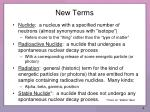New Terms
• Nuclide: a nucleus with a specified number of neutrons (almost synonymous with “isotope”)
• Refers more to the “thing” rather than the “type of matter”
• Radioactive Nuclide: a nuclide that undergoes a spontaneous nuclear decay process
• With a corresponding release of some energetic particle (or photon)
• Radiation: general (historic) term for the kind of energetic particles (or photons) that are emitted from a sample containing radioactive nuclides. Many kinds:
• Alpha, beta, gamma, positron
• Stable Nuclide*: a nuclide that does not undergo any spontaneous nuclear decay process. *more on “stable” later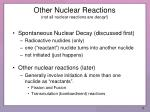• Spontaneous Nuclear Decay (discussed first)
• Radioactive nuclides (only)
• one (“reactant”) nuclide turns into another nuclide
• not initiated (just happens)
• Other nuclear reactions (later)
• Generally involve initiation & more than one nuclide as “reactants”.
• Fission and Fusion
• Transmutation (bombardment) reactions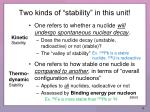Two kinds of “stability” in this unit!
• One refers to whether a nuclide will undergo spontaneous nuclear decay.
• Does the nuclide decay (unstable, radioactive) or not (stable)?
• The “valley of stability”

KineticStability

Ex. 206Pb is a stable nuclide. 238U is radioactive

• One refers to how stable one nuclide is compared to another, in terms of “overall configuration of nucleons”
• Applies to all nuclides, radioactive or not
• Assessed by Binding energy per nucleon

Thermo-dynamicStability

(later)

Ex. 56Fe is more stable than 206Pb or 2H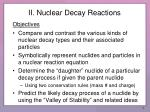II. Nuclear Decay Reactions

Objectives

• Compare and contrast the various kinds of nuclear decay types and their associated particles
• Symbolically represent nuclides and particles in a nuclear reaction equation
• Determine the “daughter” nuclide of a particular decay process if given the parent nuclide
• Using two conservation rules (mass # and charge)
• Predict the likely decay process of a nuclide by using the “Valley of Stability” and related ideas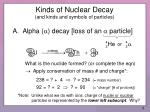Kinds of Nuclear Decay (and kinds and symbols of particles)

or

234

Th

90

What is the nuclide formed? (or complete the eqn)

 Apply conservation of mass # and charge*:

238 = ? + 4  ? = (= mass number)

234

92 = ? + 2  ? = (= “charge” [# of protons])

90

*Note: Unlike what we do with ions, charge of nuclei or nuclear particles is represented by the lower left subscript. Why?

A. Alpha (a) decay [loss of an a particle]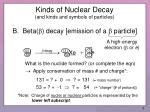Kinds of Nuclear Decay (and kinds and symbols of particles)

A high-energy electron (b or e)

0

131

Xe

-1

54

What is the nuclide formed? (or complete the eqn)

 Apply conservation of mass # and charge*:

131 = ? + 0  ? = (= mass number)

131

53 = ? + -1  ? = (= “charge” [# of protons])

54

*Note: Charge of nuclei or nuclear particles is represented by the lower left subscript.

B. Beta(b) decay [emission of a b particle]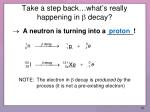Take a step back…what’s really happening in b decay?

proton

0

1

p

-1

1

0

131

Xe

-1

54

NOTE: The electron in b decay is produced by the process (it is not a pre-existing electron)

 A neutron is turning into a _______!Kinds of Nuclear Decay (What else can happen?)

C. Gamma g radiation [emission of a g particle]

A high-energy photon

• Photon = “particle” of light (no mass)
• “Repacking” of a nucleus
• An “excited” nucleus “relaxes” to lower energy, with an emission of a photon
• Not unlike an electron in an atom
• Usually happens after another nuclear reactionKinds of Nuclear Decay (What else can happen?)

C. Gamma g radiation [emission of a g particle]

A high-energy photon

• Basically, nothing appears to happen!Kinds of Nuclear Decay (What else can happen?)

A “positive electron”,

30

Si

14

What is the nuclide formed? (or complete the eqn)

 Apply conservation of mass # and charge*:

30 = ? + 0  ? = (= mass number)

30

15 = ? + 1  ? = (= “charge” [# of protons])

14

D. Positron emission [emission of a positron]0

1

n

+1

0

30

Si

14

NOTE: A proton will not decay this way spontaneously unless it is in certain nuclei. Free protons are “stable”.

 A proton is turning into a neutron!A comment on “antimatter”
• It is true that “when matter meets antimatter, they mutually annihilate one another to form pure energy”
• A positron is a type of antimatter; an electron is a type of (regular) matter.

Antimatter is real!Kinds of Nuclear Decay (Guess what? There is a second way to turn a p into a n!)
• Different than the others
• “Added particle” is a reactant(not “produced”)
• A preexisting electron (inner shell) gets “snagged” by the nucleus (?!)

7

Li

3

E. Electron CaptureNuclear Stability Patterns—The Valley of Stability
• How can we predict which kinds of nuclear decays occur in which nuclides?
• Is there a pattern?
• Yes! But let’s start by looking at which nuclides are stable.
• NOTE: I will not be explaining why these ones are stable. This is, primarily, empirical.

Ppt 06 Nuclear ChemistryNuclear Stability Patterns—The Valley of Stability
• Make a plot of number of neutrons (N) vs number of protons (Z) for stable nuclides
• This “defines” the so-called “valley of stability” (also “zone” or “belt” of stability)
• See board first. Then figures.

Ppt 06 Nuclear ChemistryTable 19.2 Number of Stable Nuclides with Even and Odd Numbers of Nucleons

Even numbers (of nucleons) appear to correlate with stability. Theory of nucleon energy levels is beyond the scope of this course (and my knowledge!)

Ppt 06 Nuclear ChemistryThe stable nuclides lie in a very narrow band of neutron-to-proton ratios.
• The ratio of neutrons to protons in stable nuclides gradually increases as the number of protons in the nucleus increases.
• Light nuclides, such as 12C, contain about the same number of neutrons and protons.
• Heavy nuclides, such as 238U, contain up to 1.6 times as many neutrons as protons.
• There are no stable nuclides with atomic numbers larger than 83.
• This narrow band of stable nuclei is surrounded by a sea of instability.
• Nuclei that lie below the band don't have enough neutrons and are therefore neutron-poor.
• Nuclei that lie above the band have too many neutrons and are therefore neutron-rich.

Ppt 06 Nuclear ChemistryNOTE:

The farther away a nuclide is from the valley of stability, the shorter its half life.

“Farther = less

(kinetically) stable”

http://en.wikipedia.org/wiki/File:Isotopes_and_half-life.svg

Ppt 06 Nuclear ChemistryMore from the web…
• http://en.wikipedia.org/wiki/Isotope
• http://atom.kaeri.re.kr/Figure 18.16 (Zumdahl) A Plot of (Potential) Energy versus the Separation Distance (particles = protons)

Ppt 06 Nuclear Chemistry

26Summary of Strategy for Predicting Decay Type

*Discussed later

• If Z > 83, predict a-decay (“Beyond”)
• Not always “correct”, but correct prediction
• Figure out if the nuclide has:
• “too many neutrons” (“Above”) OR
• “too few neutrons” (“Below”)
• (NOTE: long way or shortcut way*; even if you use shortcut, be able to relate it to the n/p ratio!)
• Make conclusion by noting which process makes daughter closer to the “valley” (next slide)Summary of Strategy for Predicting Decay Type (continued)
• It turns out that…

A radioactive nuclide tends to decay in such a way that its daughter nuclide is closer to the valley of stability

• b: turns n to p

 used by nuclides above valley (“neutron rich”)

• PE or EC: turns p to n

 used by nuclides below valley (“neutron poor”)

• a: lose both n and p

 used by nuclides beyond valley (too many of both)Summary of Strategy for Predicting Decay Type (continued)

To determine if a nuclide is “above”, “below”, or “beyond” (if you don’t have a valley of stability table):

• Long way:
• Calculate n/p (=N/Z) ratio
• Compare actual n/p ratio to ~stable n/p ratio:
• Know that for Z = 1-20, n/p = 1 is ~stable
• Know that for Z = ~80, n/p = 1.5 is ~stable
• Know that for Z ~40, n/p ~1.25 is stable
• Short way: (next slide)Summary of Strategy for Predicting Decay Type (continued)

To determine if a nuclide is “above”, “below”, or “beyond” (if you don’t have a valley of stability table):

• Short way (makes some assumptions, but…):

 Compare A/p to “aam”/p

(“aam” = average atomic mass of element)

• Why does this work?
• It is likely to be the case that the most abundant isotopes on Earth are “stable”.
• Thus, the average atomic mass, if rounded, is likely to be close to the mass number of nuclides near the valley of stability!Examples

See handout sheet and board examplesPpt 06 Nuclear ChemistryPpt 06 Nuclear ChemistryFigure 18.7 Geiger-Muller Counter

Rates of nuclear reactions (“activities”) can be directly measured using a Geiger counter.Table 19.6 Some Radioactive Nuclides, with Half-Lives and Medical Applications as Radiotracers

Ppt 06 Nuclear ChemistryEnergy-related Issues
• See Board
• Consider a nucleus of an atom of U-238
• How much mass do you think it should contain?
• mass of a proton = 1.007276 amu
• mass of a neutron = 1.008665 amu
• mass of an electron = 0.00054858 amu

Ppt 06 Nuclear Chemistry1 Mg-30 nucleus

6 He-5 nuclei

2 N-15 nuclei

3 Be-10 nuclei

Ppt 06 Nuclear ChemistryDoes it continue this way if we consider combining larger amounts of nucleons? Say, six times more (i.e., 240)?Ppt 06 Nuclear ChemistryFigure 18.10 Both Fission and Fusion CAN Produce More Stable Nuclides and are thus Exothermic

IF nuclide is very large;

(fusion of large nuclides would be endothermic!!)

IF nuclide is small. (fission of small nuclides would be endothermic!!)

Ppt 06 Nuclear ChemistryFigure 18.11 Fission

Ppt 06 Nuclear ChemistryPpt 06 Nuclear ChemistryPpt 06 Nuclear Chemistry